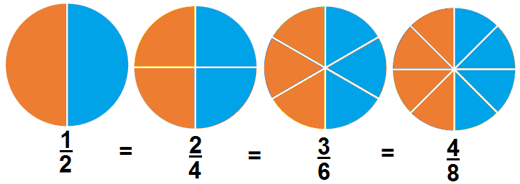# Fractions

### Decimal Fractions

A Fraction is an expression that indicates the quotient of two quantities (it is a way of representing division of a ‘whole’ into ‘parts’), such as ¾

3/4 is read as two thirds (or three quarters).

A Common Fraction is a fraction written in the form of two whole numbers, one above the other, separated by a line.

The numerator tells how many parts are being considered. The denominator tells the total number of equal parts (the whole).

Identify fractions on number linesEquivalent FractionsTwo fractions are equivalent if they represent the same number.

Equivalent fractions are fractions that may look different, but are equal to each other.

### Equivalent Fractions

You can convert all fractions to decimals dividing the numerator by the denominator.

1/2= 0.5

2/4 = 0.5

3/6 = 0.5

4/8 = 0.5

We can use cross multiplication to decide whether two fractions are equivalent.Cross-Multiplying means that we multiply the numerator of each side by the denominator of the other side.### FR09      Fractions_Lengths_Word Problems

Like Fractions Help

Your ability to understand math problems can be improved with math journal writing. Be sure to include all the relevant information.   Remember- Show your work and answers in Your Math Journal!

Create a graphic organizer (Frayer Model) for the following vocabulary words: Fraction, Numerator, Denominator, Proper Fraction, Improper Fraction, Mixed Number, Simplest Form, Equivalent Fraction.

Fractions in real life situations:

I have two pizzas, and I share them equally between three people. How much will they each get?